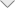### Analysis of RCS Problems in Ship

Introduction

The radar range equation expresses the range at which a target may be detected with a given probability by a radar having a given set of parameters. This equation includes the target radar cross section (RCS), which is a measure of the proportion of the incident energy reflected back to the radar. This returned energy varies with a multitude of parameters such as transmitted wavelength, target geometry, orientation, and reflectivity. The radar cross section of an object is proportional to the far-field ratio of reflected to incident power density, that is:

Radar cross section can be efficiently estimated using PO solution (ray-tracing algorithm) in EMC Studio and EMCoS Antenna VLab environment.

Simulation Model Description

This application note demonstrates calculation of Radar Cross Section of ship and comparison with analytical solution and RCS typical values.Typical Values of RCS for Ships

RCS of ship depends on overall size and Gross/displacement tonnage of ship. Typical values for ships are known and described below: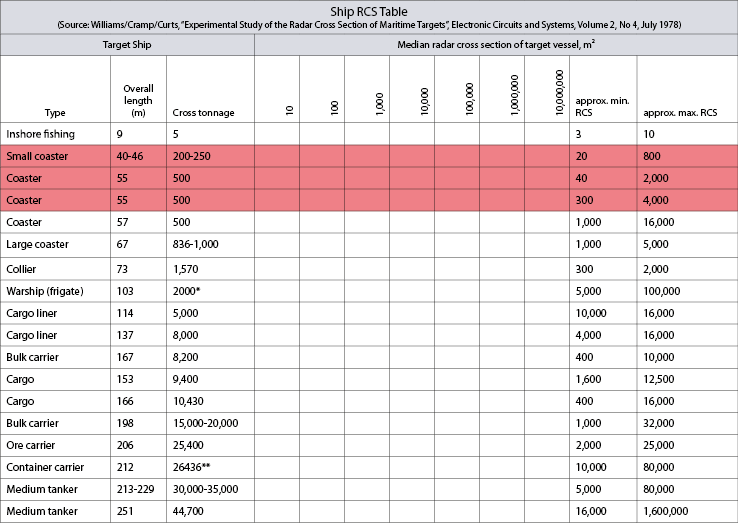Median values for ship with approximate tonnage from 200-500 t are from 13 dBsm to 36 dBsm (highlighted rows).

Analytic Prediction of Radar Cross Section

Results calculated from empirical expression are in very good agreement with measured data. Analytical solution can be efficiently used for validation of simulation results.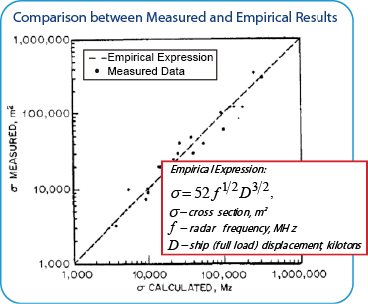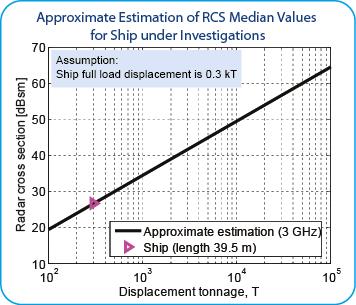Results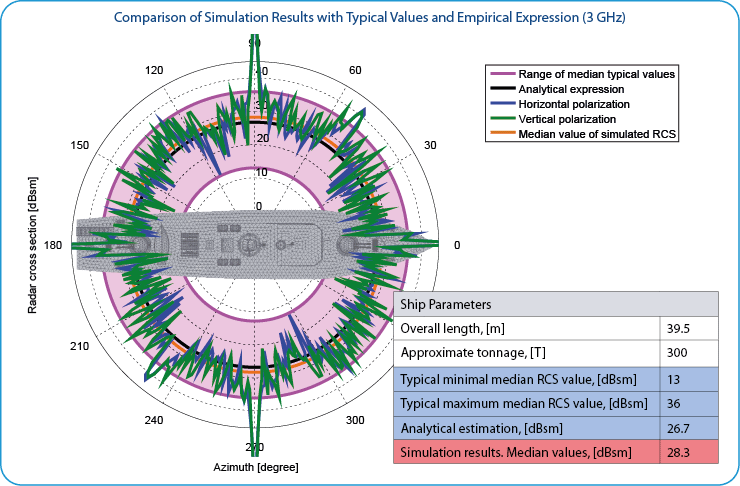From the obtained results it can be seen that simulation results are in very good agreement with typical RCS values and analytical expression.

Conclusions

According to performed investigation the main conclusions are:

• EMC Studio and EMCoS Antenna VLab provide the powerful tools for analysis of Radar Cross Section problems in ship
• For calculation of radar cross section PO solution (ray-tracing algorithm) is considered
• Simulation results were compared with typical RCS values and approximate analytical solution

References

• Skolnik in Eustace, H.F. (Ed.). The international Countermeasures Handbook, 3rd ed., pp. 278-279, 1977, 78. Courtesy Cardiff Publ. Co.
• Williams/Cramp/Curts, ‘Experimental Study of the Radar Cross Section of Maritime Targets’, Electronic Circuits and Systems, Volume 2, No 4, July 1978
﻿